Next: 5.2.3 Random Background Charge Up: 5.2.2 Error Rate/Probability Previous: Bit Errors

### Read/Write Errors and Access Time

Due to the stochastic nature of the tunnel process, the exact tunnel time of an electron is not known. One only knows rates and probabilities, which results in the need to wait considerably longer than the actual charging process would take, to assure with a certain error probability, that an electron actually tunneled. From (2.23) it follows that the tunnel rate will be of the magnitude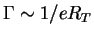. Thus, the average tunnel time is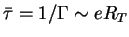. With a typical tunnel resistance of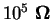the average tunnel time becomes0.02 ps. If one assumes that a tera-bit (1012) chip is written or read every second for three years (108 s) and one permits in average only one error, one needs an error probability for reading and writing of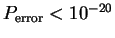. Assuming an exponential distribution of the tunnel time (3.28) of an electron gives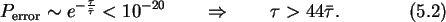Therefore one must wait about 1 ps for an electron to tunnel with an error rate lower than 10-20. One picosecond would still be a very good access time. But the values used for this estimation can easily vary by a factor ten or even hundred. For instance, a tunnel resistance of 1 M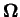is a common value. This would raise the estimated value to 10 ps. Equally, the assumption of a free energy drop of 1 eV, which was used for the rate estimation, for the tunnel event might not be reached. A smaller change in free energy reduces the tunnel rate and prolongs the average tunnel time.Next: 5.2.3 Random Background Charge Up: 5.2.2 Error Rate/Probability Previous: Bit Errors

Christoph Wasshuber